## Cut Set Matrix in Network Analysis:

A connected graph can be separated into two parts by removing certain branches of the graph. This is equivalent to cutting a graph into two parts hence it is refered as Cut Set Matrix in Network Analysis.

A cut-set is a minimal set of branches of a connected graph such that after removal of these branches graph gets separated into two distinct parts, each of which a connected graph, with the condition that replacing any one branch from the cutset makes the graph connected.

Consider an oriented graph as shown in the Fig. 5.14 (a).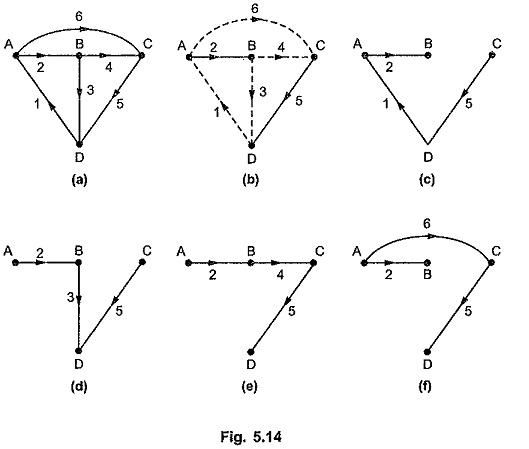Consider that branches 1, 3, 4 and 6 are removed from graph which are shown by dashed lines in the Fig. 5.14 (b). The graph is divided into two parts each of which is connected. Now each branch is replaced one at a time, we get connected graph for every branch replaced as shown in the Fig. 5.14 (c), (d), (e) and (f). Thus a set of branches [1, 3, 4, 5] forms a cut-set. We can have another set of branches [6, 2, 3, 5] giving another cutset. Hence for any graph, there may be more than one cutsets and every graph has at least one cut-set.

The cut-set separates the nodes of the graph into 2 group each being in one of the two parts. So the branch of cut-set has one of its terminals incident at a node in one group and its other end at a node in the other group. So we can consider that all the branches joined at a node forms a cut-set since by removing them, the graph would be splitted into two parts.

### Cut-Set Matrix (Qa):

The Cut Set Matrix in Network Analysis Qa can be written by considering the orientation of the cut-set from one of the two parts to the other. Consider an oriented graph as shown in the Fig. 5.15 (a). Consider orientations of cut-sets arbitrarily. The possible 6 cut-sets are as shown in the Fig. 5.15 (b).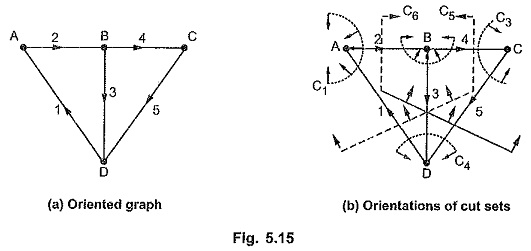The elements of cut-set matrix Qa are given as follows,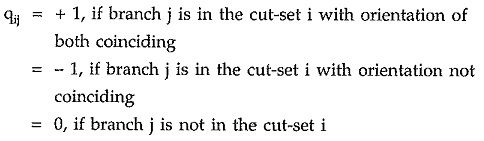For the graph shown in the Fig. 5.15 (b), the cut-sets can be listed as follows,

Cut-set – C1 : [1, 2]

Cut-set – C2 : [2, 3, 4]

Cut-set – C3 : [4, 5]

Cut-set – C4 : [1, 3, 5]

Cut-set – C5 : [1, 3, 4]

Cut-set – C6 : [2, 3, 5]

The Cut Set Matrix in Network Analysis can be written tabular form,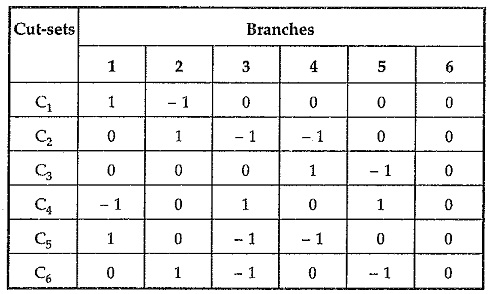Thus, cutset matrix Qa is given by,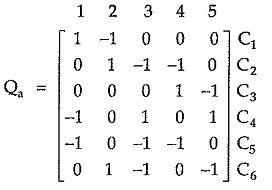For any graph, number of cut-sets may be extensively large so to ease simplification subset of cut-set matrix is considered which is called fundamental cut-set or f-cut-set matrix.

### Fundamental Cut-set (or f-cut-set Matrix) (Q):

A fundamental cut-set of a graph is a set of one twig and chords at a node. It is apparent that at each node for each twig, there will be a fundamental cut-set. For a graph with n nodes, there will be n – 1 number of fundamental cut-sets.

The orientation of f-cutsets is selected such that it coincides with the orientation of the defining twig of f cutset.

Consider an oriented graph as shown in the Fig. 5.16 (a). Select one tree as shown in the Fig. 5.16 (b). The twigs are shown by dark lines while links are shown by dashed lines. The fundamental cut-sets are given by (n – 1) i.e. (4 – 1) = 3. The fundamental cut-sets are as shown in the Fig. 5.16 (c), (d), and (e).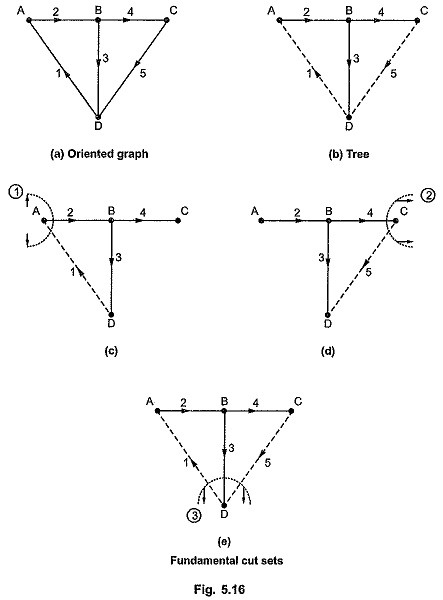The f-cutsets can be listed as below.

f – cut-set 1 : [2, 1]

f – cut-set 2 : [4, 5]

f – cut-set 3 : [3, 5, 1]

The f-cut-set matrix can be written matrix form as follows in which writing twigs entries first, then links entries.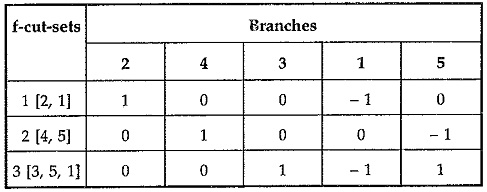Hence the fundamental cut-set matrix Q is given by,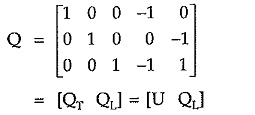where QT is the twig matrix which is the identity matrix while QL is the link matrix.

Scroll to Top